# RS Aggarwal Solutions for Class 8 Maths Chapter 3 - Square and Square Roots Exercise 3C

RS Aggarwal Solutions for Class 8 Maths Chapter3 Exercise 3C are provided here. The questions present in this exercise have been solved by BYJU’S subject experts, and this will help students in solving the questions without any obstacles. The solutions prepared are according to the RS Aggarwal Solutions guidelines to understand each and every concept. Exercise 3C of class 8 helps students to understand the concepts of column method as well as the diagonal method for squaring a two-digit number. By practising the RS Aggarwal Solutions for class 8, students will be able to grasp the concepts perfectly. Here students will get the solutions of each and every question with an explanation by BYJU’S experts.

## Download PDF of RS Aggarwal Solutions for Class 8 Maths Chapter 3 – Squares and Square Roots – Exercise 3C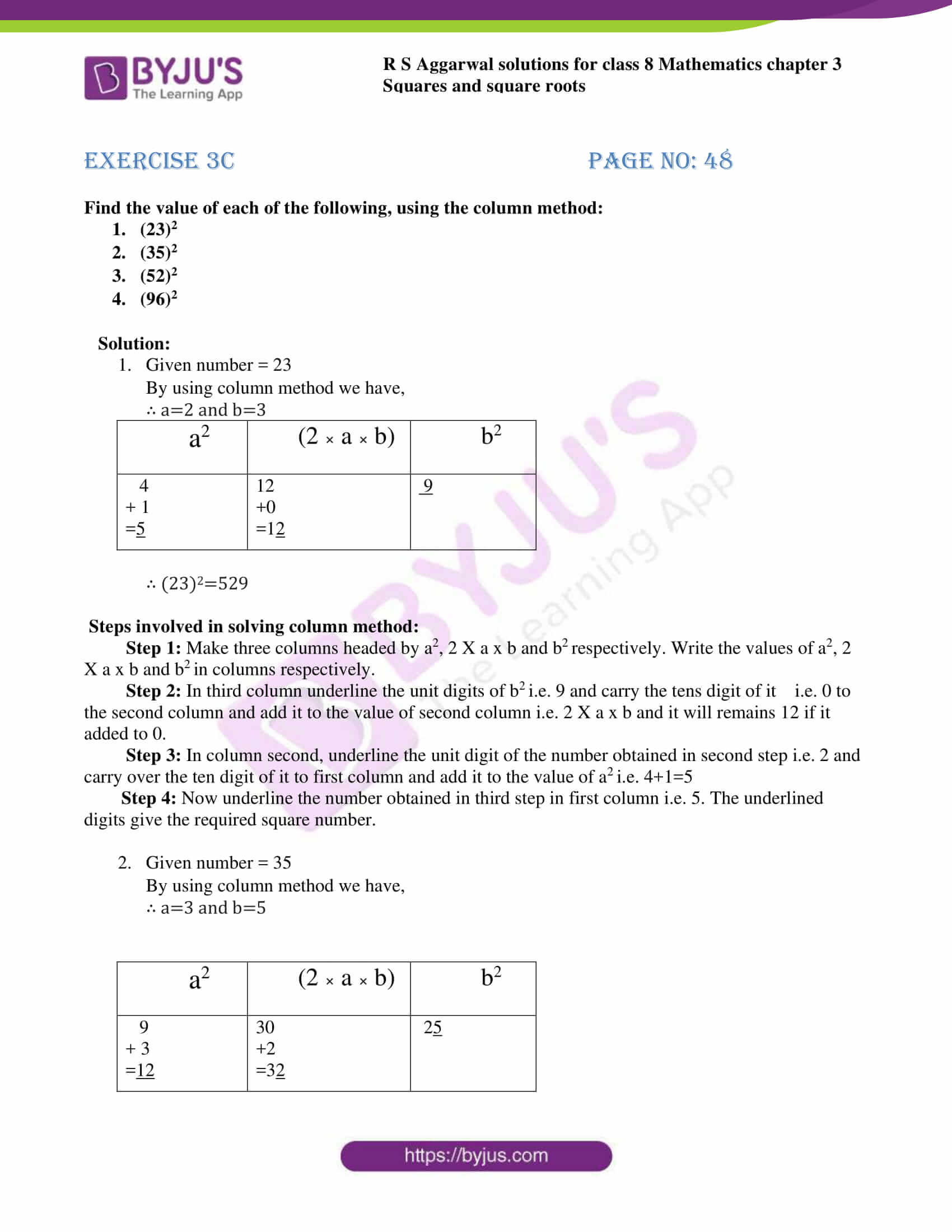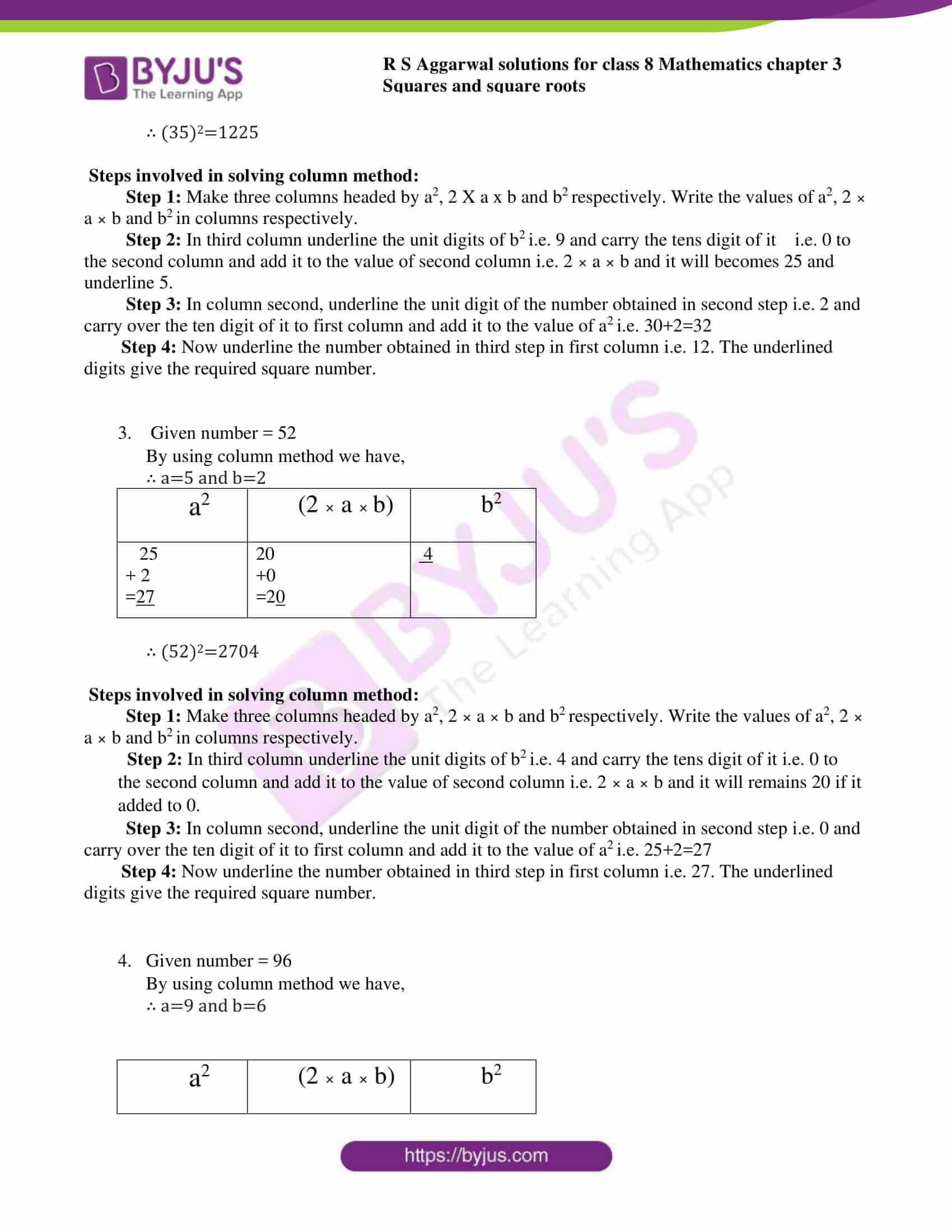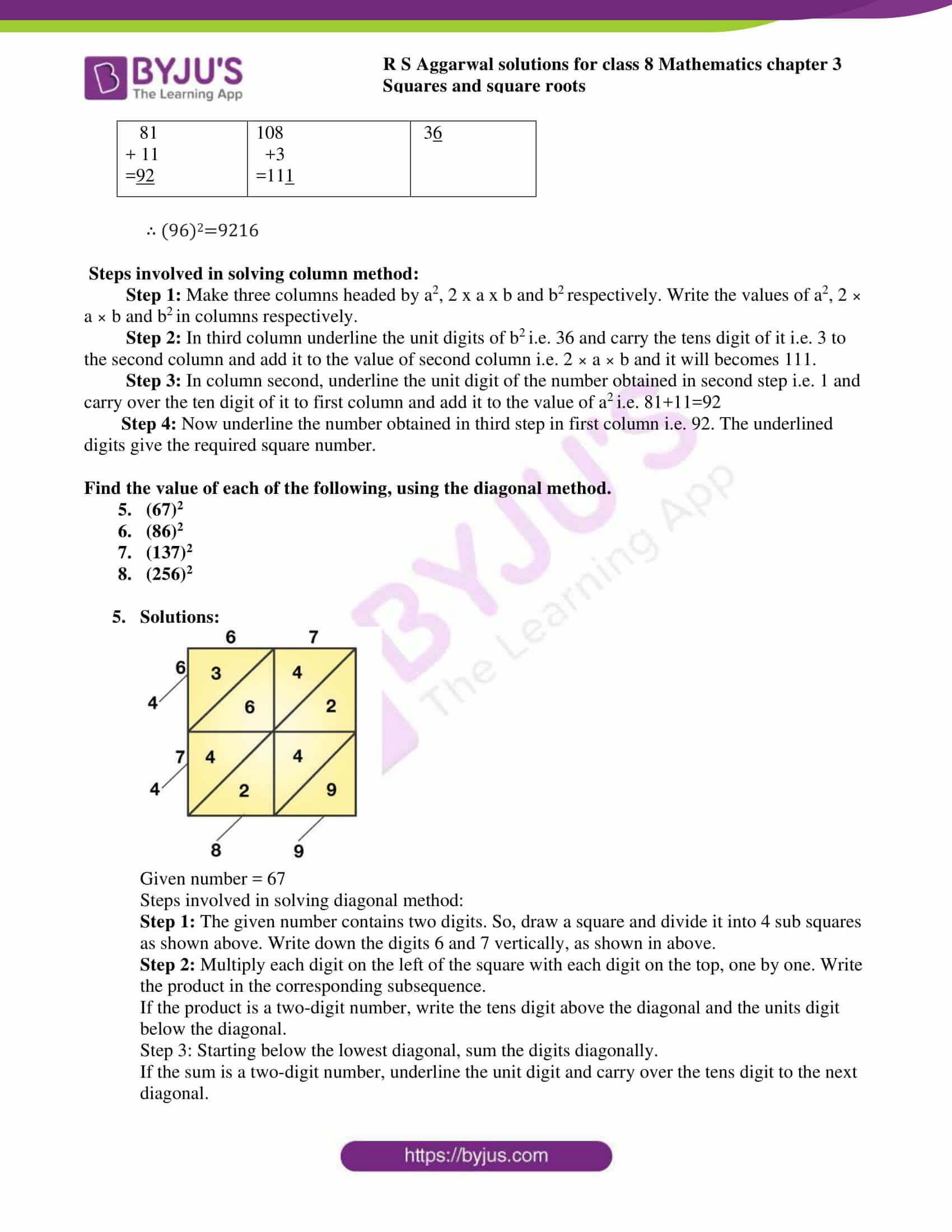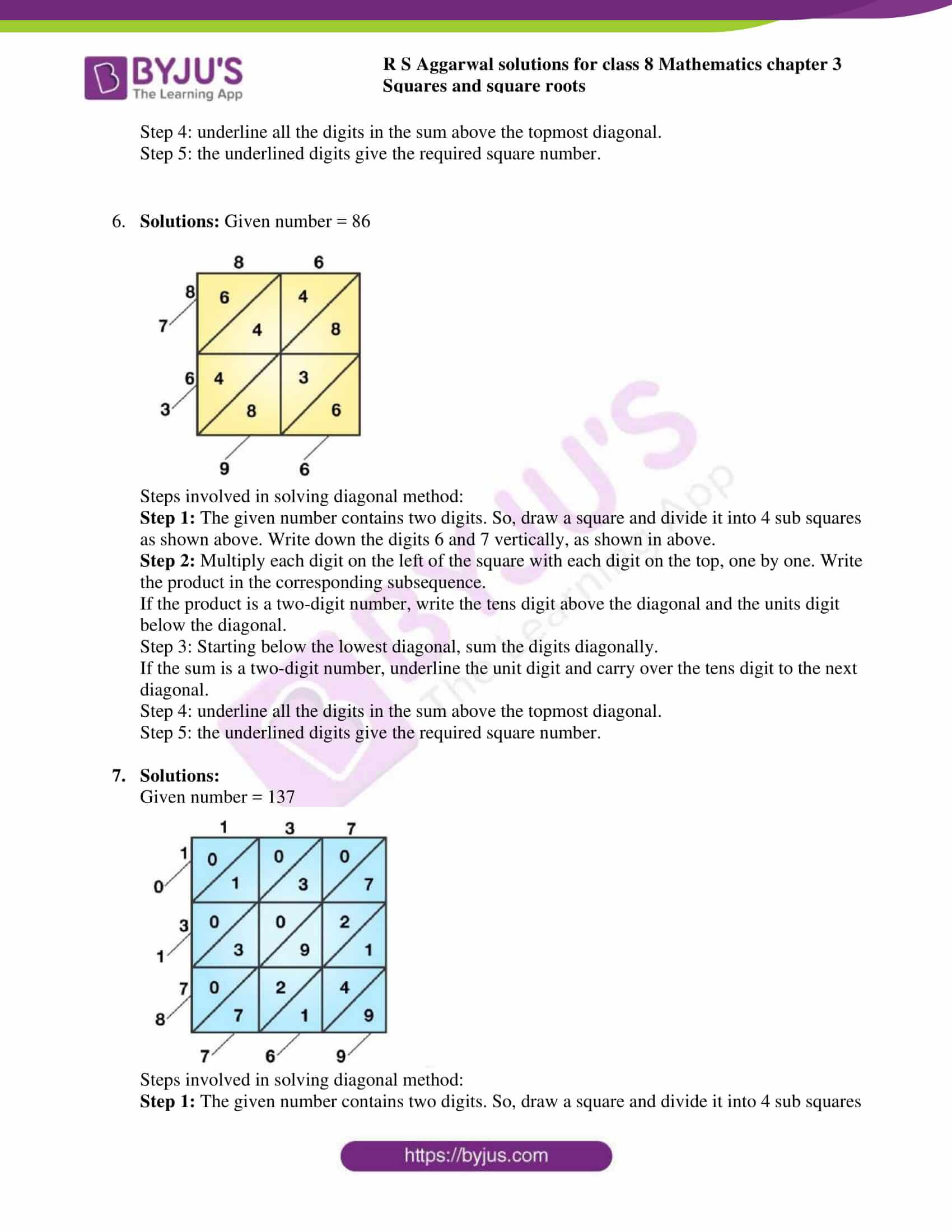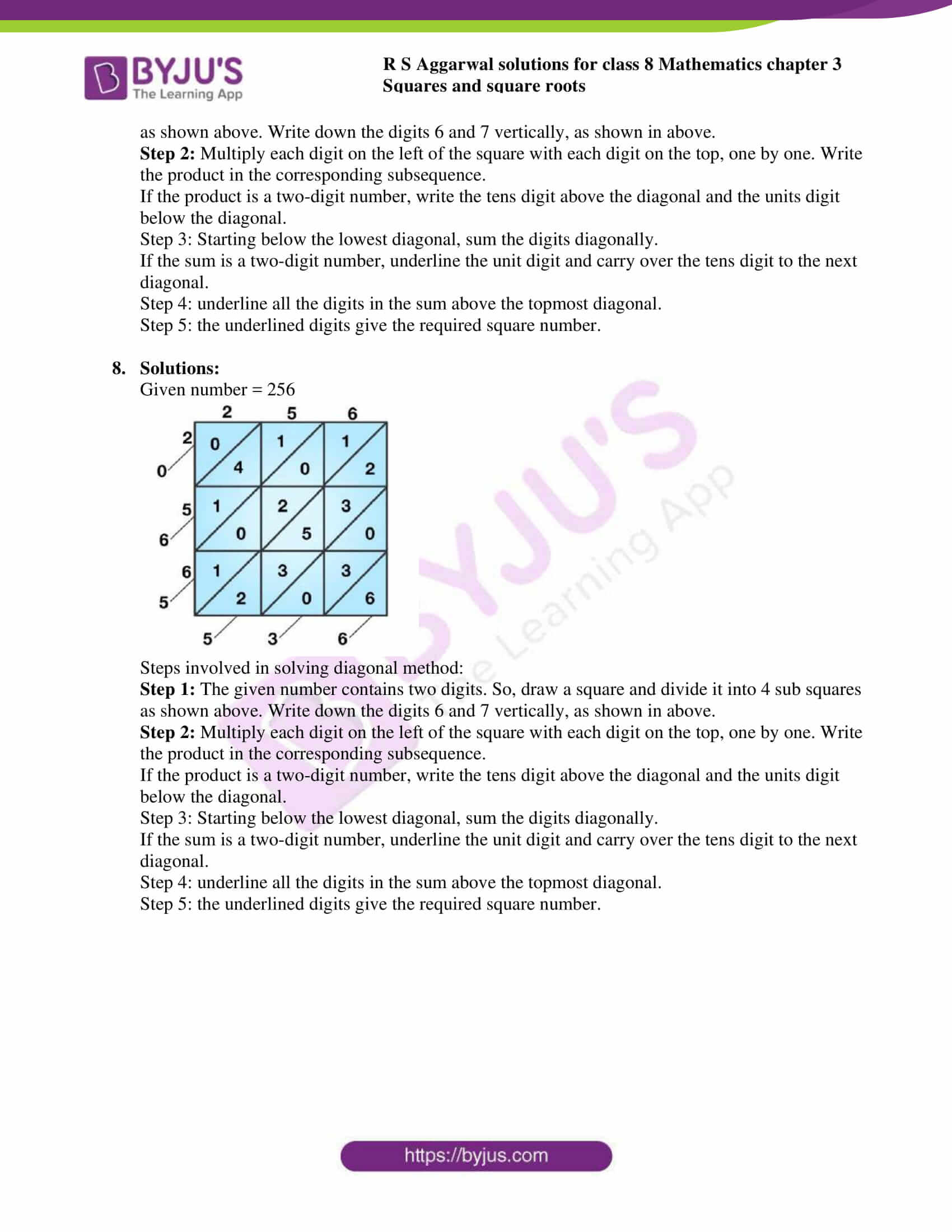## Exercise 3C

Find the value of each of the following, using the column method:

1. (23)2

2. (35)2

3. (52)2

4. (96)2

Solution:

1. Given number = 23By using column method we have,

∴ a=2 and b=3

 a2 (2 × a × b) b2 4 + 1 =5 12 +0 =12 9

∴ (23)2=529

Steps involved in solving column method:

Step 1: Make three columns headed by a2, 2 X a x b and b2 respectively. Write the values of a2, 2 X a x b and b2 in columns respectively.

Step 2: In third column underline the unit digits of b2 i.e. 9 and carry the tens digit of it i.e. 0 to the second column and add it to the value of second column i.e. 2 X a x b and it will remains 12 if it added to 0.

Step 3: In column second, underline the unit digit of the number obtained in second step i.e. 2 and carry over the ten digit of it to first column and add it to the value of a2 i.e. 4+1=5

Step 4: Now underline the number obtained in third step in first column i.e. 5. The underlined digits give the required square number.

2. Given number = 35By using column method we have,

∴ a=3 and b=5

 a2 (2 × a × b) b2 9 + 3 =12 30 +2 =32 25

∴ (35)2=1225

Steps involved in solving column method:

Step 1: Make three columns headed by a2, 2 X a x b and b2 respectively. Write the values of a2, 2 × a × b and b2 in columns respectively.

Step 2: In third column underline the unit digits of b2 i.e. 9 and carry the tens digit of it i.e. 0 to the second column and add it to the value of second column i.e. 2 × a × b and it will becomes 25 and underline 5.

Step 3: In column second, underline the unit digit of the number obtained in second step i.e. 2 and carry over the ten digit of it to first column and add it to the value of a2 i.e. 30+2=32

Step 4: Now underline the number obtained in third step in first column i.e. 12. The underlined digits give the required square number.

3. Given number = 52By using column method we have,

∴ a=5 and b=2

 a2 (2 × a × b) b2 25 + 2 =27 20 +0 =20 4

∴ (52)2=2704

Steps involved in solving column method:

Step 1: Make three columns headed by a2, 2 × a × b and b2 respectively. Write the values of a2, 2 × a × b and b2 in columns respectively.

Step 2: In third column underline the unit digits of b2 i.e. 4 and carry the tens digit of it i.e. 0 to the second column and add it to the value of second column i.e. 2 × a × b and it will remains 20 if it added to 0.

Step 3: In column second, underline the unit digit of the number obtained in second step i.e. 0 and carry over the ten digit of it to first column and add it to the value of a2 i.e. 25+2=27

Step 4: Now underline the number obtained in third step in first column i.e. 27. The underlined digits give the required square number.

4. Given number = 96By using column method we have,

∴ a=9 and b=6

 a2 (2 × a × b) b2 81 + 11 =92 108 +3 =111 36

∴ (96)2=9216

Steps involved in solving column method:

Step 1: Make three columns headed by a2, 2 x a x b and b2 respectively. Write the values of a2, 2 × a × b and b2 in columns respectively.

Step 2: In third column underline the unit digits of b2 i.e. 36 and carry the tens digit of it i.e. 3 to the second column and add it to the value of second column i.e. 2 × a × b and it will becomes 111.

Step 3: In column second, underline the unit digit of the number obtained in second step i.e. 1 and carry over the ten digit of it to first column and add it to the value of a2 i.e. 81+11=92

Step 4: Now underline the number obtained in third step in first column i.e. 92. The underlined digits give the required square number.

Find the value of each of the following, using the diagonal method.

5. (67)2

6. (86)2

7. (137)2

8. (256)2

1. Solutions: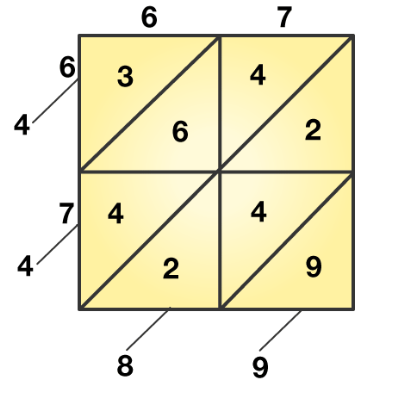Given number = 67

Steps involved in solving diagonal method:

Step 1: The given number contains two digits. So, draw a square and divide it into 4 sub squares as shown above. Write down the digits 6 and 7 vertically, as shown in above.

Step 2: Multiply each digit on the left of the square with each digit on the top, one by one. Write the product in the corresponding subsequence.

If the product is a two-digit number, write the tens digit above the diagonal and the units digit below the diagonal.

Step 3: Starting below the lowest diagonal, sum the digits diagonally.

If the sum is a two-digit number, underline the unit digit and carry over the tens digit to the next diagonal.

Step 4: underline all the digits in the sum above the topmost diagonal.

Step 5: the underlined digits give the required square number.

2. Solutions: Given number = 86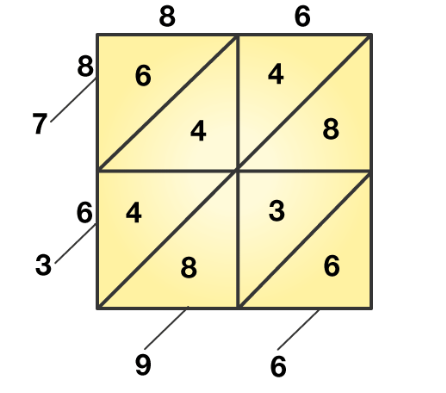Steps involved in solving diagonal method:

Step 1: The given number contains two digits. So, draw a square and divide it into 4 sub squares as shown above. Write down the digits 6 and 7 vertically, as shown in above.

Step 2: Multiply each digit on the left of the square with each digit on the top, one by one. Write the product in the corresponding subsequence.

If the product is a two-digit number, write the tens digit above the diagonal and the units digit below the diagonal.

Step 3: Starting below the lowest diagonal, sum the digits diagonally.

If the sum is a two-digit number, underline the unit digit and carry over the tens digit to the next diagonal.

Step 4: underline all the digits in the sum above the topmost diagonal.

Step 5: the underlined digits give the required square number.

3. Solutions: Given number = 137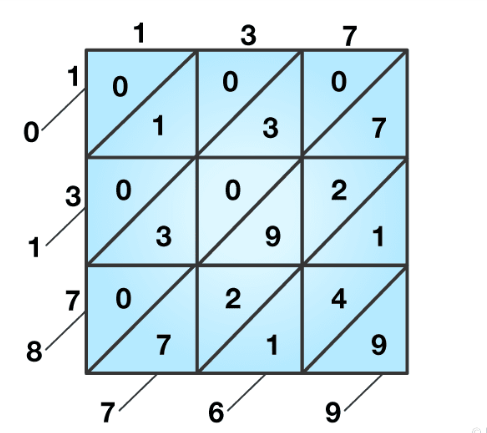Steps involved in solving diagonal method:

Step 1: The given number contains two digits. So, draw a square and divide it into 4 sub squares as shown above. Write down the digits 6 and 7 vertically, as shown in above.

Step 2: Multiply each digit on the left of the square with each digit on the top, one by one. Write the product in the corresponding subsequence.

If the product is a two-digit number, write the tens digit above the diagonal and the units digit below the diagonal.

Step 3: Starting below the lowest diagonal, sum the digits diagonally.

If the sum is a two-digit number, underline the unit digit and carry over the tens digit to the next diagonal.

Step 4: underline all the digits in the sum above the topmost diagonal.

Step 5: the underlined digits give the required square number.

4. Solutions: Given number = 256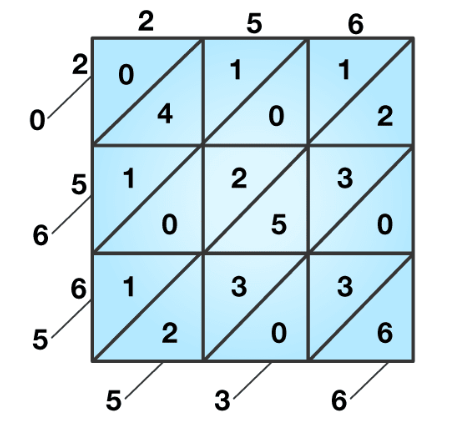Steps involved in solving diagonal method:

Step 1: The given number contains two digits. So, draw a square and divide it into 4 sub squares as shown above. Write down the digits 6 and 7 vertically, as shown in above.

Step 2: Multiply each digit on the left of the square with each digit on the top, one by one. Write the product in the corresponding subsequence.

If the product is a two-digit number, write the tens digit above the diagonal and the units digit below the diagonal.

Step 3: Starting below the lowest diagonal, sum the digits diagonally.

If the sum is a two-digit number, underline the unit digit and carry over the tens digit to the next diagonal.

Step 4: underline all the digits in the sum above the topmost diagonal.

Step 5: the underlined digits give the required square number.

### Access other exercises of RS Aggarwal Solutions for Class 8 Maths Chapter 3 – Squares and Square Roots

Exercise 3A Solutions 6 questions

Exercise 3B Solutions 13 questions

Exercise 3D Solutions 18 questions

Exercise 3E Solutions 19 questions

Exercise 3F Solutions 12 questions

Exercise 3G Solutions 10 questions

Exercise 3H Solutions 19 questions

## RS Aggarwal Solutions for Class 8 Maths Chapter 3 – Squares and Square Roots Exercise 3C

Exercise 3C of RS Aggarwal Solutions for Chapter 3, Squares and Square Roots, contains 8 questions, which are based on column method and diagonal method. Exercise 3C of RS Aggarwal Solutions for Chapter 3, Squares and Square Roots deals with the basic concepts related to methods for squaring a two-digit number. We can say that this exercise mainly deals with the methods for squaring two-digit numbers such as column method and diagonal method that the students have learnt in this chapter. Some of the topics focused prior to exercise 3C include the following.

Column method for squaring a two-digit number

• Diagonal method for squaring a two-digit number

The RS Aggarwal Solutions can help the students in practising and learning each and every concept as it provides solutions to all questions asked in RS Aggarwal textbook.